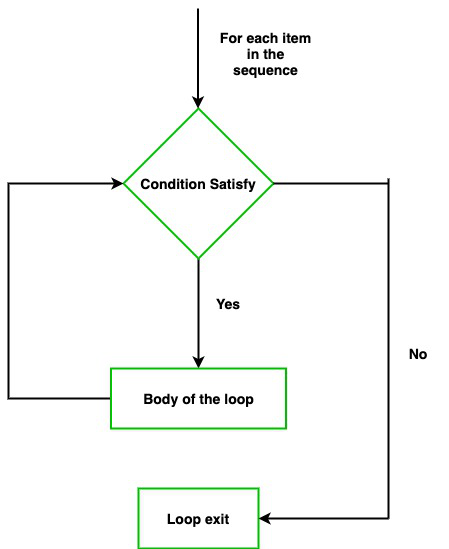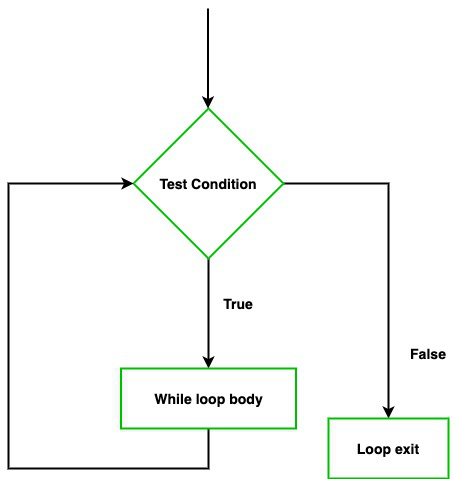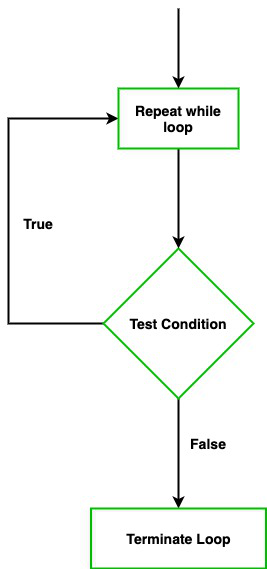# Swift – Loops

• Last Updated : 05 Dec, 2021

In general, a loop is used for iteration and iteration means repetition. Using loops we can do anything n number of times. A loop can iterate infinite times until the condition fails. To break the loop generally, we write a condition. For example, if we want to print Hello GeeksforGeeks 100 times we use a loop, now we have to specify a condition n<=100 kinds of thing in the loop. If the iteration is 101 the loop breaks.

Types of loops in swift

• for-in loop
• while loop
• repeat while loop

Let us know about each loop separately along with syntax and examples.

### for-in loop

Generally for-in loop and if conditions are very similar to each other. If condition is used to execute the block of code when the specified condition is satisfied but it is executed only once. Using a for-in loop we can execute the same code n number of times. It is used to execute the block of code whenever the condition is satisfied and when the condition fails the loop will break. for-in loop in swift supports many ways to use a for-in loop. We can iterate over elements in arrays, sets, dictionaries, etc. And we can use for loop with range, range with where clause. And we can also use the for-in loop with stride() function. Let us see the syntax and flow diagram of for loop.

Syntax:

for item in range

{

// Statements

}

Flow Chart:Example:

## Swift

 `// Swift program to illustrate the use of for-in loop`` ` `// Creating and initializing variables``var` `a = 6, sum1 = 0, i = 0`` ` `// Finding perfect number``for` `i ``in` `1...a-1``{``    ``if` `(a % i == 0)``    ``{``        ``sum1 += i``    ``}``}`` ` `// Checking if the sum is``// equal to a or not``if` `(sum1 == a)``{``    ``print``(``"Given number is a perfect number"``)``}``else``{``    ``print``(``"given number is not a perfect number"``)``}`

Output:

`Given number is a perfect number`

Explanation: In the above example, we have declared the required variables. Then using for loop we have written logic for perfect number. Then using the if statement we are checking whether the sum1 value and a value are the same. If both values are equal we are just printing it is a perfect number Otherwise we are printing it is not a perfect number .

### While loop

While loop is used to execute a piece of code inside its block until the condition is satisfied. Once the condition is failed the loop will break. This is also similar to for loop. But in for loop, we write initialization, condition, and increment/decrement in for loop itself. In while loop before writing while block we initialize the iteration variables and all. Inside the while loop we write condition and increment/decrement statements. While loop is also called the pre-test loop. This means the condition is checked before entering into the loop. If the condition is satisfied then only it will enter into the while block.

Syntax:

while condition

{

// Statements

increment/decrement

}

Flow diagram:Example:

## Swift

 `// Swift program to illustrate the use of while loop`` ` `// Creating and initializing variables``var` `a = 5, fact = 1, i = 1`` ` `// Finding the factorial ``// Using while loop``while` `(i <= a)``{``    ``fact *= i``    ``i += 1``}``print``(``"Factorial of given number is"``, fact)`

Output:

`Factorial of given number is 120`

Explanation: In the above example, we have declared the required variables using the var keyword. In the next step, we have used a while loop and iterating over a given range. In the while loop, we have written the logic for factorial numbers. Finally printing the fact variable which holds the value of factorial of given range.

### Repeat – while loop

Repeat – while loop is very similar to do-while loop in C programming. The do-while block is executed once before checking the condition. This means the block is executed at least once even if the condition fails in the first iteration. Likewise in repeat – while loop the block is executed once even if the condition fails in the first iteration. Let us see the syntax for a repeat while loop. The syntax is also similar to the do-while loop in C language. Repeat – while loop is also called an exit-controlled loop.

Syntax:

repeat

{

// Statements

}while(condition)

Flow diagram:Example:

## Swift

 `// Swift program to illustrate the use of repeat-while loop`` ` `// Creating and initializing variables``var` `a = 10``var` `i = 1`` ` `// Using repeat-while loop``repeat``{``    ``print``(i)``    ``i = i + 1``}``while``(i > a)`

Output:

`1`

Explanation: In the above example, first we have declared the required variables. In the next step, we have initialized repeat while loop. And we have written some logic for printing every element in a given range inside the loop. Finally, we are writing while followed by the condition. Here only one printed because the condition is failed in the first iteration itself. But we got 1 as output because since it is a repeat – while loop. The block is executed at least 1 time. So that we got 1 as output.

My Personal Notes arrow_drop_up